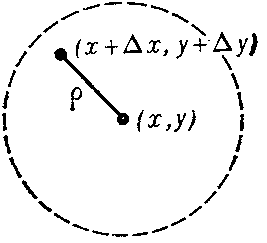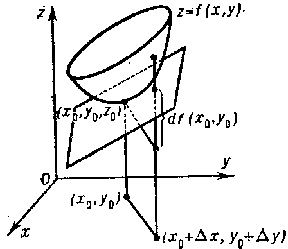# Differential

The main linear part of increment of a function.

1) A real-valued function $f$ of a real variable $x$ is said to be differentiable at a point $x$ if it is defined in some neighbourhood of this point and if there exists a number $A$ such that the increment

$$\Delta y = f ( x + \Delta x ) - f ( x)$$

may be written (if the point $x + \Delta x$ lies in this neighbourhood) in the form

$$\Delta y = A \Delta x + \omega ,$$

where $\omega / \Delta x \rightarrow 0$ as $\Delta x \rightarrow 0$. Here $A \Delta x$ is usually denoted by $dy$ and is called the differential of $f$ at $x$. For a given $x$ the differential $dy$ is proportional to $\Delta x$, i.e. is a linear function of $\Delta x$. By definition, as $\Delta x \rightarrow 0$ the additional term $\omega$ is infinitely small of a higher order than $\Delta x$( and also than $dy$ if $A \neq 0$). This is why the differential is said to be the main part of increment of the function.

For a function which is differentiable at a point $x$, $\Delta y \rightarrow 0$ if $\Delta x \rightarrow 0$, i.e. a function which is differentiable at a point is continuous at that point. A function $f$ is differentiable at a point $x$ if and only if it has, at that point, a finite derivative

$$f ^ { \prime } ( x) = \lim\limits _ {\Delta x \rightarrow 0 } \ \frac{\Delta y }{\Delta x } = A ;$$

moreover,

$$d y = f ^ { \prime } ( x) \Delta x .$$

There exist continuous functions which are not differentiable.

The designation $df ( x)$ may be used instead of $dy$, and the above equation assumes the form

$$d f ( x) = f ^ { \prime } ( x) \Delta x .$$

The increment of the argument $\Delta x$ is then usually denoted by $dx$, and is said to be the differential of the independent variable. Accordingly, one may write

$$d y = f ^ { \prime } ( x) d x .$$

Hence $f ^ { \prime } ( x) = dy / dx$, i.e. the derivative is equal to the ratio of the differentials $dy$ and $dx$. If $A \neq 0$, then $\Delta y / dy \rightarrow 1$ as $\Delta x \rightarrow 0$, i.e. if $A \neq 0$, then $\Delta y$ and $dy$ are infinitesimals of the same order as $\Delta x \rightarrow 0$; this fact, along with the simple structure of the differential (i.e. linearity with respect to $\Delta x$), is often used in approximate computations, by assuming that $\Delta y \approx dy$ for small $\Delta x$. E.g., if it is desired to compute $f ( x + \Delta x )$ from a known $f ( x)$ when $\Delta x$ is small, it is assumed that

$$f ( x + \Delta x ) \approx f( x) + dy .$$

Obviously, such reasoning is useful only if it is possible to estimate the magnitude of the error involved.

Geometric interpretation of the differential. The equation of the tangent to the graph of a function $f$ at a point $( x _ {0} , y _ {0} )$ is of the form $y - y _ {0} = f ^ { \prime } ( x _ {0} ) ( x - x _ {0} )$. If one puts $x = x _ {0} + \Delta x$, then $y - y _ {0} = f ^ { \prime } ( x _ {0} ) \Delta x$. The right-hand side represents the value of the differential of the function $f$ at the point $x _ {0}$ corresponding to the value of $\Delta x$ being considered. Thus, the differential is identical with the corresponding increment of the ordinate of the tangent to the curve $y = f ( x)$( cf. the segment $NT$ in Fig. a). Here $\omega = \Delta y - dy$, i.e. the value of $| \omega |$ coincides with the length of the segment $TS$.Figure: d031810a

2) The definitions of differentiability and differential are readily extended to real-valued functions of $n$ real variables. Thus, in the case $n = 2$ a real-valued function is said to be differentiable at a point $( x , y )$ with respect to both variables $x$ and $y$ if it is defined in some neighbourhood of this point and if its total increment

$$\Delta z = f ( x + \Delta x , y + \Delta y ) - f ( x , y )$$

may be written as

$$\Delta z = A \Delta x + B \Delta y + \alpha ,$$

where $A$ and $B$ are real numbers, $\alpha / \rho \rightarrow 0$ if $\rho \rightarrow 0$, $\rho = \sqrt {\Delta x ^ {2} + \Delta y ^ {2} }$; it is assumed that the point $( x + \Delta x , y + \Delta y )$ belongs to the neighbourhood mentioned above (Fig. b).Figure: d031810b

One introduces the notation

$$d z = d f ( x , y ) = A \Delta x + B \Delta y;$$

$dz$ is said to be the total differential, or simply the differential, of the function $f$ at the point $( x , y )$( the phrase "with respect to both variables x and y" is sometimes added). For a given point $( x , y )$ the differential $dz$ is a linear function of $\Delta x$ and $\Delta y$; the difference $\alpha = \Delta z - dz$ is infinitely small of a higher order than $\rho$. In this sense $dz$ is the main linear part of the increment $\Delta z$.

If $f$ is differentiable at the point $( x , y )$, then it is continuous at this point and has finite partial derivatives (cf. Derivative)

$$f _ {x} ^ { \prime } ( x , y ) = A ,\ \ f _ {y} ^ { \prime } ( x , y ) = B$$

at this point. Thus

$$d z = d f ( x , y ) = f _ {x} ^ { \prime } ( x , y ) \Delta x + f _ {y} ^ { \prime } ( x , y ) \Delta y .$$

The increments $\Delta x$ and $\Delta y$ of the independent variables are usually denoted by $dy$ and $dx$, as in the case of a single variable. One may write, accordingly,

$$d z = d f ( x , y ) = f _ {x} ^ { \prime } ( x , y ) d x + f _ {y} ^ { \prime } ( x , y ) d y .$$

The existence of finite partial derivatives does not, in general, entail the differentiability of the function (even if it is assumed to be continuous).

If a function $f$ has a partial derivative with respect to $x$ at a point $( x , y )$, the product $f _ {x} ^ { \prime } ( x , y ) dx$ is said to be its partial differential with respect to $x$; in the same manner, $f _ {y} ^ { \prime } ( x , y ) dy$ is the partial differential with respect to $y$. If the function is differentiable, its total differential is equal to the sum of the partial differentials. Geometrically, the total differential $df ( x _ {0} , y _ {0} )$ is the increment in the $z$- direction in the tangent plane to the surface $z = f ( x , y )$ at the point $( x _ {0} , y _ {0} , z _ {0} )$, where $z _ {0} = f ( x _ {0} , y _ {0} )$( Fig. c).Figure: d031810c

The following is a sufficient criterion for the differentiability of a function: If in a certain neighbourhood of a point $( x _ {0} , y _ {0} )$ a function $f$ has a partial derivative $f _ {x} ^ { \prime }$ which is continuous at $( x _ {0} , y _ {0} )$ and, in addition, has a partial derivative $f _ {y} ^ { \prime }$ at that point, then $f$ is differentiable at that point.

If a function $f$ is differentiable at all points of an open domain $D$, then at any point of the domain

$$d z = A ( x , y ) d x + B ( x , y ) d y ,$$

where $A ( x , y ) = f _ {x} ^ { \prime } ( x , y )$, $B ( x , y ) = f _ {y} ^ { \prime } ( x , y )$. If, in addition, there exist continuous partial derivatives $A _ {y} ^ \prime$ and $B _ {x} ^ { \prime }$ in $D$, then, everywhere in $D$,

$$A _ {y} ^ \prime = B _ {x} ^ { \prime } .$$

This proves, in particular, that not every expression

$$A ( x , y ) d x + B ( x , y ) d y$$

with continuous $A$ and $B$( in a domain $D$) is the total differential of some function of two variables. This is a difference from functions of one variable, where any expression $A ( x) d x$ with a continuous function $A$ in some interval is the differential of some function.

The expression $A dx + B dy$ is the total differential of some function $z = f ( x , y )$ in a simply-connected open domain $D$ if $A$ and $B$ are continuous in this domain, meet the condition $A _ {y} ^ \prime = B _ {x} ^ { \prime }$ and, in addition: a) $A _ {y} ^ \prime$ and $B _ {x} ^ { \prime }$ are continuous or b) $A$ and $B$ are everywhere differentiable in $D$ with respect to both variables $x$ and $y$, .

See also Differential calculus for differentials of real-valued functions of one or more real variables and for differentials of higher orders.

3) Let a function $f$ be defined on some set $E$ of real numbers, let $x$ be a limit point of this set, let $x \in E$, $x + \Delta x \in E$, $\Delta y = A \Delta x + \alpha$, where $\alpha / \Delta x \rightarrow 0$ if $\Delta x \rightarrow 0$; then the function $f$ is called differentiable with respect to the set $E$ at $x$, while $dy = A \Delta x$ is called its differential with respect to the set $E$ at $x$. This is a generalization of the differential of a real-valued function of one real variable. Special kinds of this generalization include differentials at the end points of the interval within which the function is defined, and the approximate differential (cf. Approximate differentiability).

Differentials with respect to a set for real-valued functions of several real variables are introduced in a similar manner.

4) All definitions of differentiability and a differential given above can be extended, almost unchanged, to complex-valued functions of one or more real variables; to real-valued and complex-valued vector-functions of one or more real variables; and to complex functions and vector-functions of one or more complex variables. In functional analysis they are extended to functions of the points of an abstract space. One may speak of differentiability and of the differential of a set function with respect to some measure.

How to Cite This Entry:
Differential. Encyclopedia of Mathematics. URL: http://encyclopediaofmath.org/index.php?title=Differential&oldid=53429
This article was adapted from an original article by G.P. Tolstov (originator), which appeared in Encyclopedia of Mathematics - ISBN 1402006098. See original article